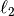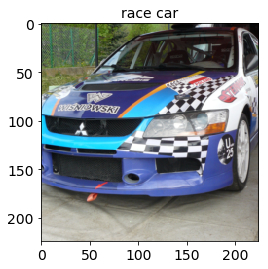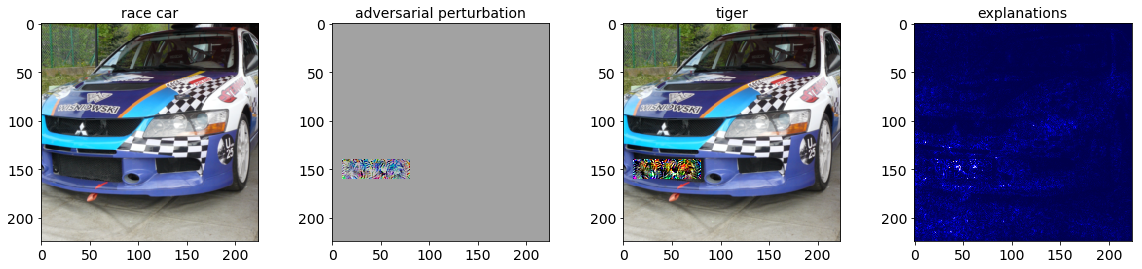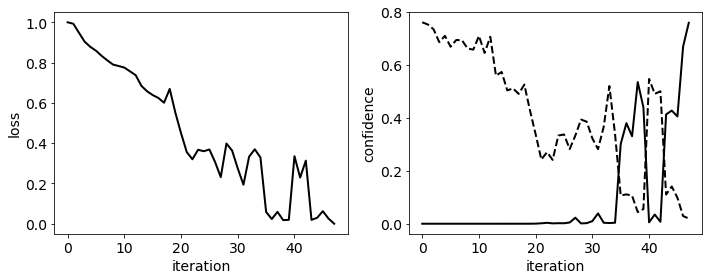# 2.8. Evasion Attacks on ImageNet (Advanced)¶

We show here how to run different evasion attacks against ResNet-18, a DNN pretrained on ImageNet. This notebook enables running also CleverHans attacks (implemented in TensorFlow) against PyTorch models.

We aim to have the image of a race car misclassified as a tiger, using the-norm targeted implementations of the Carlini-Wagner (CW) attack (from CleverHans), and of our PGD attack.

We also consider a variant of our PGD attack, referred to as PGD-patch, where we restrict the attacker to only change the pixels of the image corresponding to the license plate, using a box constraint (see Melis et al., Is Deep Learning Safe for Robot Vision? Adversarial Examples against the iCub Humanoid, ICCVW ViPAR 2017, https://arxiv.org/abs/1708.06939).

We start by loading the pre-trained ResNet18 model from torchvision, and we pass it to the SecML wrapper. Then, we load the ImageNet labels.

:

# NBVAL_IGNORE_OUTPUT
from torchvision import models

model = models.resnet18(pretrained=True)

:

import io

import numpy as np
import requests
import torch

from secml.ml.classifiers import CClassifierPyTorch
from secml.ml.features import CNormalizerMeanStd

# Set random seed for pytorch and numpy
np.random.seed(0)
torch.manual_seed(0)

# imagenet normalization
normalizer = CNormalizerMeanStd(mean=(0.485, 0.456, 0.406),
std=(0.229, 0.224, 0.225))

# wrap the model, including the normalizer
clf = CClassifierPyTorch(model=model,
input_shape=(3, 224, 224),
softmax_outputs=False,
preprocess=normalizer,
random_state=0,
pretrained=True)

import json

imagenet_labels_path = "https://raw.githubusercontent.com/" \
"anishathalye/imagenet-simple-labels/" \
"master/imagenet-simple-labels.json"
r = requests.get(imagenet_labels_path)


We load the image of a race car that we would like to have misclassified as a tiger and we show it, along with the classifier prediction.

:

import numpy as np
from PIL import Image
from torchvision import transforms

from secml.array import CArray

transform = transforms.Compose([
transforms.Resize(256),
transforms.CenterCrop(224),
transforms.ToTensor(),
])

img_path = "https://images.pexels.com/photos/589782/pexels-photo-589782.jpeg"\
"?cs=srgb&dl=car-mitshubishi-race-rally-589782.jpg&fm=jpg"
r = requests.get(img_path)
img = Image.open(io.BytesIO(r.content))

# apply transform from torchvision
img = transform(img)
# transform the image into a vector
img = torch.unsqueeze(img, 0).view(-1)
img = CArray(img.numpy())

# get the classifier prediction
preds = clf.predict(img)
pred_class = preds.item()
pred_label = labels[pred_class]

# create a function to show images
def plot_img(f, x, label):
x = np.transpose(x.tondarray().reshape((3, 224, 224)), (1, 2, 0))
f.sp.title(label)
f.sp.imshow(x)
return f

# show the original image
from secml.figure import CFigure
# Only required for visualization in notebooks
%matplotlib inline

fig = CFigure(height=4, width=4, fontsize=14)
plot_img(fig, img, label=pred_label)
fig.show()## 2.8.2. Run the attack¶

We should create the attack class that we will use to compute the attack. Changing the value of the variable attack_type, you can choose between three different attacks: CW, PGD, and PGD-patch.

Note that the PGD-patch attack may take some time to run depending on the machine.

:

attack_type = 'PGD-patch'
# attack_type = 'PGD'
# attack_type = 'CW'


To perform a PGD-patch attack that only manipulates the license plate in our car image, we need to define a proper box constraint along with its upper and lower bounds. To this end, we create the following function:

:

def define_lb_ub(image, x_low_b, x_up_b, y_low_b, y_up_b, low_b, up_b, n_channels=3):

# reshape the img (it is stored as a flat vector)
image = image.tondarray().reshape((3, 224, 224))

# assign to the lower and upper bound the same values of the image pixels
low_b_patch = deepcopy(image)
up_b_patch = deepcopy(image)

# for each image channel, set the lower bound of the pixels in the
# region defined by x_low_b, x_up_b, y_low_b, y_up_b equal to lb and
# the upper  bound equal to up in this way the attacker will be able
# to modify only the pixels in this region.
for ch in range(n_channels):
low_b_patch[ch, x_low_b:x_up_b, y_low_b:y_up_b] = low_b
up_b_patch[ch, x_low_b:x_up_b, y_low_b:y_up_b] = up_b

return CArray(np.ravel(low_b_patch)), CArray(np.ravel(up_b_patch))


We instantiate and run the attack, and show the resulting adversarial image along with its explanations, computed via integrated gradients.

:

from copy import deepcopy

from cleverhans.attacks import CarliniWagnerL2

from secml.data import CDataset

lb = 0
ub = 1
target_idx = 292  # tiger

attack_id = ''
attack_params = {}

if attack_type == "CW":
attack_id = 'e-cleverhans'
attack_params = {'max_iterations': 50, 'learning_rate': 0.005,
'binary_search_steps': 1, 'confidence': 1e6,
'abort_early': False, 'initial_const': 0.4,
'n_feats': 150528, 'n_classes': 1000,
'y_target': target_idx,
'clip_min': lb, 'clip_max': ub,
'clvh_attack_class': CarliniWagnerL2}

if attack_type == 'PGD':
attack_id = 'e-pgd'
solver_params = {
'eta': 1e-2,
'max_iter': 50,
'eps': 1e-6}
attack_params = {'distance': 'l2',
'dmax': 1.875227,
'lb': lb,
'ub': ub,
'y_target': target_idx,
'solver_params': solver_params}

if attack_type == 'PGD-patch':
attack_id = 'e-pgd'
# create the mask that we will use to allows the attack to modify only
# a restricted region of the image
x_lb = 140; x_ub = 160; y_lb = 10; y_ub = 80
dmax_patch = 5000
lb_patch, ub_patch = define_lb_ub(
img, x_lb, x_ub, y_lb, y_ub, lb,ub, n_channels=3)
solver_params = {
'eta': 0.8,
'max_iter': 50,
'eps': 1e-6}

attack_params = {'distance': 'l2',
'dmax': dmax_patch,
'lb': lb_patch,
'ub': ub_patch,
'y_target': target_idx,
'solver_params': solver_params}

val = CDataset(img, pred_class)

attack = CAttackEvasion.create(
attack_id,
clf,
surrogate_classifier=clf,
surrogate_data=val,
**attack_params)

# run the attack
eva_y_pred, _, eva_adv_ds, _ = attack.run(img, pred_class)

# get the classifier prediction


We compute the explanations for the adversarial image with respect to the target class and visualize the attack results. See the Explainable Machine Learning tutorial for more information.

:

# compute the explanations w.r.t. the target class

fig = CFigure(height=4, width=20, fontsize=14)

fig.subplot(1, 4, 1)
# plot the original image
fig = plot_img(fig, img, label=pred_label)

# normalize perturbation for visualization
diff_img -= diff_img.min()
diff_img /= diff_img.max()

fig.subplot(1, 4, 2)
fig = plot_img(fig, diff_img, label='adversarial perturbation')

fig.subplot(1, 4, 3)

fig.subplot(1, 4, 4)

expl = np.transpose(expl.tondarray().reshape((3, 224, 224)), (1, 2, 0))
r = np.fabs(expl[:, :, 0])
g = np.fabs(expl[:, :, 1])
b = np.fabs(expl[:, :, 2])

# Calculate the maximum error for each pixel
expl = np.maximum(np.maximum(r, g), b)
fig.sp.title('explanations')
fig.sp.imshow(expl, cmap='seismic')

fig.show()## 2.8.3. Visualize and check the attack optimization¶

To check if the attack has properly converged to a good local minimum, we plot how the loss and the predicted confidence values of the target (solid line) and true class (dotted line) change across the attack iterations.

:

from secml.ml.classifiers.loss import CSoftmax
from secml.ml.features.normalization import CNormalizerMinMax

n_iter = attack.x_seq.shape
itrs = CArray.arange(n_iter)

# create a plot that shows the loss and the confidence during the attack iterations
# note that the loss is not available for all attacks
fig = CFigure(width=10, height=4, fontsize=14, linewidth=2)

# apply a linear scaling to have the loss in [0,1]
loss = attack.f_seq
if loss is not None:
loss = CNormalizerMinMax().fit_transform(CArray(loss).T).ravel()
fig.subplot(1, 2, 1)
fig.sp.xlabel('iteration')
fig.sp.ylabel('loss')
fig.sp.plot(itrs, loss, c='black')

# classify all the points in the attack path
scores = clf.predict(attack.x_seq, return_decision_function=True)

# we apply the softmax to the score to have value in [0,1]
scores = CSoftmax().softmax(scores)

fig.subplot(1, 2, 2)
fig.sp.xlabel('iteration')
fig.sp.ylabel('confidence')
fig.sp.plot(itrs, scores[:, pred_class], linestyle='--', c='black')
fig.sp.plot(itrs, scores[:, target_idx], c='black')

fig.tight_layout()
fig.show()